Select Page

# MCQ Application of Derivatives CBSE Maths 12 Science Answers in English

MCQ Application of Derivatives CBSE Maths 12 Science Answers in English to enable students to get Answers in a narrative video format for the specific question.

Expert Teacher provides MCQ Application of Derivatives CBSE Maths 12 Science Answers through Video Answers in English language. This video solution will be useful for students to understand how to write an answer in exam in order to score more marks. This teacher uses a narrative style for a question from Application of Derivatives not only to explain the proper method of answering question, but deriving right answer too.

Please find the question below and view the Answer in a narrative video format.

Question:

## Similar Questions from CBSE, 12th Science, Maths, Application of Derivatives

Question 1 : Find the maximum profit that a company can make if the profit function is given by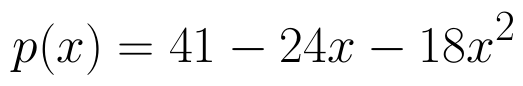(View Answer Video)

Question 2 : The maximum value of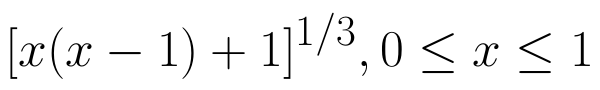is (View Answer Video)

Question 3 : The point on the curve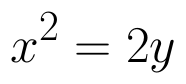which is nearest to the point (0, 5) is  (View Answer Video)

Question 4 : Find the maximum value of in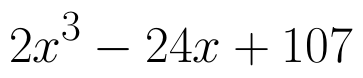the interval [1,3]. find the maximum value of the same function in [-3,-1]. (View Answer Video)

Question 5 : The normal  to the curve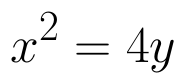passing (1,2) is____________. (View Answer Video)

### Inverse Trigonometric Functions

Question 1 : Write the principal value of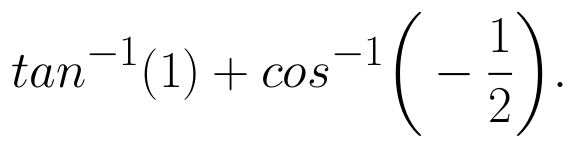(View Answer Video)

Question 2 : Solve for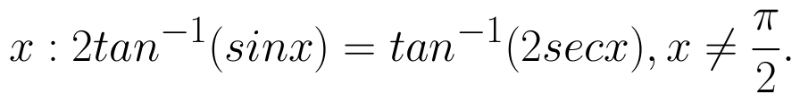(View Answer Video)

Question 3 : Write the value of the following: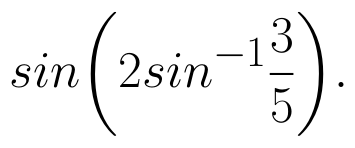(View Answer Video)

Question 4 : If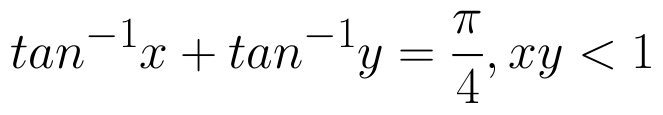, then write the value of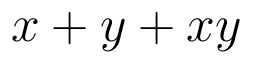. (View Answer Video)

Question 5 : Solve for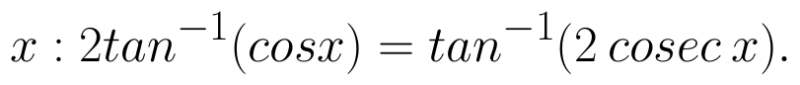(View Answer Video)

### Integrals

Question 1 : Find the integral of the function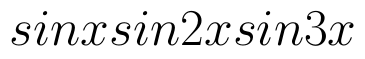. (View Answer Video)

Question 2 : Evaluate :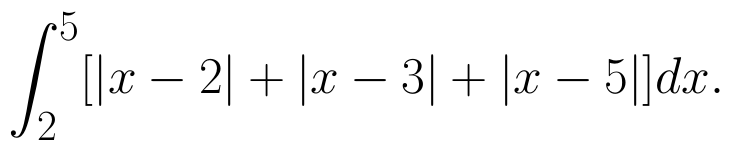(View Answer Video)

Question 3 : Evaluate :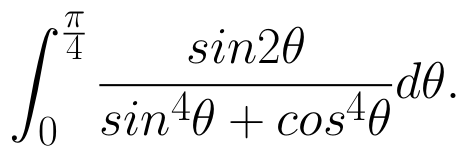(View Answer Video)

Question 4 :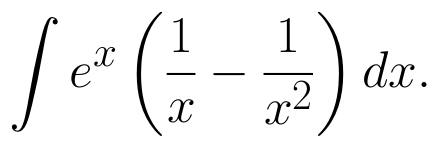(View Answer Video)

Question 5 : Evaluate :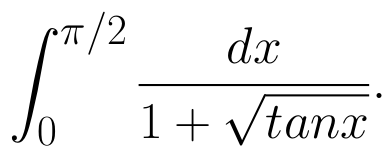(View Answer Video)

### Relations and Functions

Question 1 : If f(x) = |x| and g(x) = | 5x – 2 |. Then, fog = _____. (View Answer Video)